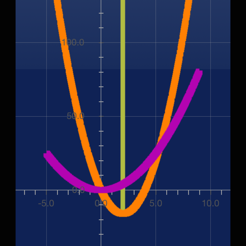## Screenshots

•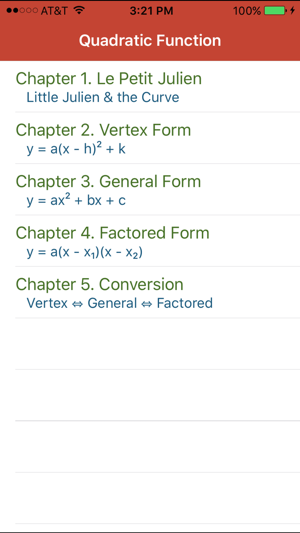•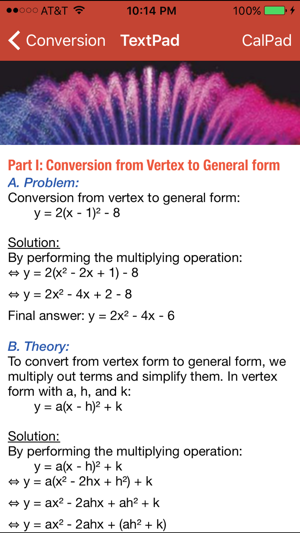•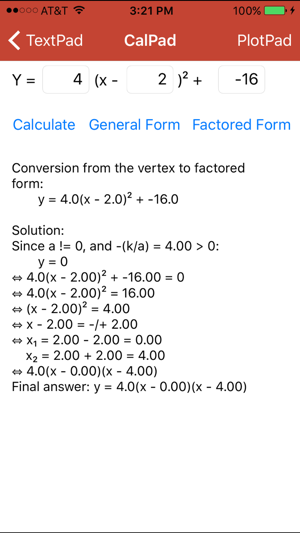•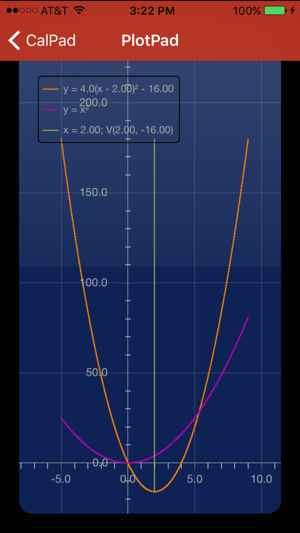•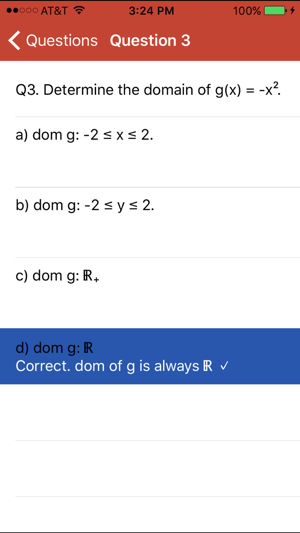## Description

This app is an interactive graphing math book in which high school, college students and science lovers can observe and try out various ways of analyzing a quadratic function with graphing features.

Students are gradually introduced the theory behind a quadratic function with various parameters. Mathematically, a quadratic function may be expressed in one of the three forms: the vertex form, the general form, and the factored form. This app shows techniques to find the zeros, the coordinates of the vertex, the y-intercept; to draw the corresponding parabola and its axis of symmetry; as well as to algebraically convert from one form to another. There are formula-specific calculators (CalPad) and parabola plotter (PlotPad) that help students characterize a quadratic function using domain, range, and variations; and visualizing the relative size and shape of the transformed parabola compared to the basic one.

• The vertex form: f(x) = a(x - h)² + k

• The general form: f(x) = ax² + bx + c

• The factored form: f(x) = a(x - x₁)(x - x₂)

## What’s New

Version 3.6

* Minor bug fixed.

* Sửa chữa một vài lỗi nhỏ.

## Information

Seller
Thinh Le
Size
14.6 MB
Category
Education
Compatibility

Requires iOS 9.3 or later. Compatible with iPhone, iPad, and iPod touch.

Languages

English

Age Rating
12+
Infrequent/Mild Sexual Content and Nudity
Price
\$0.99

## Supports

•